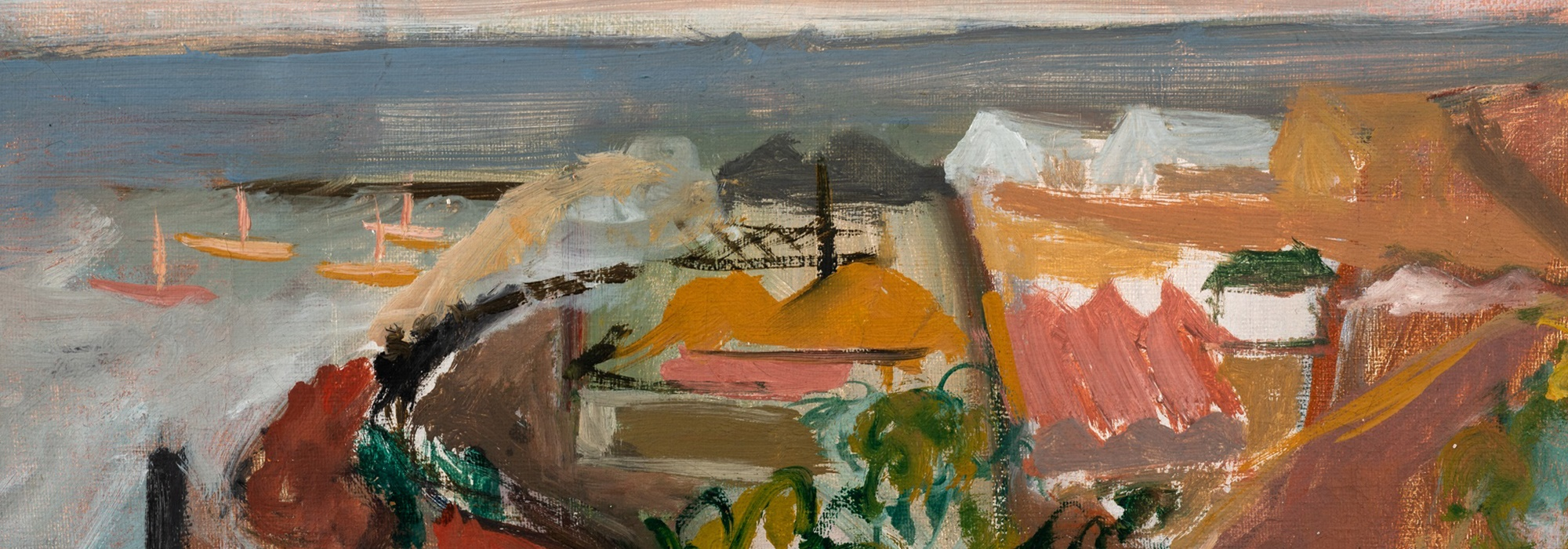##### Painting
starts: 19th Oct, 2023 11:00
Ends: 31st Oct, 2023 18:00
Location: Online

Name: Ms Carina Jansen
Email: carina@aspireart.net
Telephone: +27 78 968 2476

Name: Ms Mtha Poni
Email: mtha@aspireart.net
Telephone: +27 21 418 0765

Category

Sold for R23,450

Estimated at R15,000 - R20,000

Sold for R17,588

Estimated at R15,000 - R20,000

Sold for R9,966

Estimated at R10,000 - R15,000

Sold for R6,449

Estimated at R1,500 - R2,500

Estimated at R18,000 - R24,000

Estimated at R28,000 - R32,000

Estimated at R12,000 - R14,000

Sold for R9,380

Estimated at R10,000 - R15,000

Estimated at R7,000 - R10,000

Estimated at R20,000 - R30,000

Estimated at R20,000 - R30,000

Estimated at R30,000 - R40,000

Estimated at R20,000 - R30,000

Estimated at R40,000 - R60,000

Sold for R3,518

Estimated at R4,000 - R6,000

Sold for R21,105

Estimated at R18,000 - R24,000

Sold for R21,105

Estimated at R20,000 - R30,000

Estimated at R15,000 - R20,000

Sold for R11,725

Estimated at R8,000 - R10,000

Sold for R35,175

Estimated at R30,000 - R40,000

Sold for R14,070

Estimated at R12,000 - R18,000

Sold for R5,394

Estimated at R5,000 - R7,000

Estimated at R30,000 - R40,000

Sold for R18,760

Estimated at R18,000 - R24,000

Sold for R5,276

Estimated at R7,000 - R10,000

Sold for R12,898

Estimated at R10,000 - R15,000

Estimated at R7,000 - R10,000

Sold for R19,932

Estimated at R8,000 - R12,000

Estimated at R12,000 - R16,000

Sold for R5,394

Estimated at R5,000 - R7,000

Estimated at R100,000 - R150,000

Estimated at R50,000 - R70,000

Sold for R37,520

Estimated at R15,000 - R20,000

Sold for R17,588

Estimated at R15,000 - R20,000

Sold for R17,588

Estimated at R15,000 - R20,000

Sold for R32,830

Estimated at R30,000 - R40,000

Sold for R8,794

Estimated at R8,000 - R12,000

Estimated at R30,000 - R40,000

Estimated at R80,000 - R120,000

Estimated at R25,000 - R35,000

Sold for R56,280

Estimated at R60,000 - R80,000

Sold for R35,175

Estimated at R30,000 - R40,000

Sold for R9,380

Estimated at R6,000 - R9,000

Estimated at R20,000 - R30,000

Estimated at R15,000 - R20,000

Sold for R35,175

Estimated at R40,000 - R60,000

Sold for R19,932

Estimated at R16,000 - R18,000

Sold for R8,208

Estimated at R7,000 - R10,000